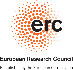# DIMEA Days 2019 - Abstracts of the talks

#### Marthe Bonamy (LaBRI, Bordeaux): Around Brooks' theorem

In this talk, we will discuss various results around Brooks' theorem: a graph has chromatic number at most its maximum degree, unless it is a clique or an odd cycle. We will consider stronger variants and local versions, as well as the structure of the solution space of all corresponding colorings.

#### Sergio Cabello (University of Ljubljana): Interactions between geometry, graphs and algorithms

I will describe some of the interactions between graphs and geometry, many of them with an algorithmic slant. In particular, I will discuss classical optimization problems for geometric intersection graphs and how tools from computational geometry become useful for some algorithmic problems on graphs.

#### Johannes Carmesin (University of Birmingham): Embedding simply connected 2-complexes in 3-space

A classical theorem of Kuratowski characterises graphs embeddable in the plane by two obstructions. More precisely, a graph is planar if and only if it does not contain the complete graph K5 or the complete bipartite graph K3,3 as a minor. Can you characterise embeddability of 2-dimensional simplicial complexes in 3-space in a way analogous to Kuratowski’s characterisation of graph planarity?

#### Zdeněk Dvořák (Charles University, Prague): Fractional chromatic number of triangle-free graphs

We will survey a number of interesting old and new results regarding the fractional chromatic number in classes of triangle-free graphs.

#### Michał Pilipczuk (University of Warsaw): A structural theory for classes of sparse graphs

The theory of structural sparsity, introduced by Nešetřil and Ossona de Mendez, is a rapidly developing area within graph theory. The goal is to develop robust notions of sparseness for graphs, based on a structural viewpoint, and to study connections between them. As such, the theory provides a wealth of tools for combinatorial and algorithmic treatment of sparse graphs, as well as uncovers deep

#### Jozef Skokan (LSE): Cycle-complete Ramsey numbers

The Ramsey number r(Cl,Kn) is the smallest natural number N such that every red/blue edge-colouring of a clique of order N contains a red cycle of length l or a blue clique of order n. In 1978, Erdős, Faudree, Rousseau and Schelp conjectured that r(Cl,Kn)=(l-1)(n-1)+1 for l≥n≥3 provided (l,n)≠(3,3). In this talk I will discuss a recent proof of this conjecture for large l, and a strong form of a conjecture due to Nikiforov, showing that r(Cl,Kn)=(l-1)(n-1)+1 provided l≥(C log n)/(log log n), for some absolute constant C>0. Up to the value of C this is tight, and answers two further questions of Erdős et al. up to multiplicative constants.

Based on joint work with Peter Keevash and Eoin Long.

#### Martin Škoviera (Comenius University, Bratislava): Cyclic connectivity, edge-elimination, and the twisted Isaacs graphs

Edge-elimination is an operation of removing an edge together with its end-vertices. We study the effect of this operation on the cyclic connectivity of a cubic graph. Disregarding a small number of cubic graphs with no more than six vertices, this operation cannot decrease cyclic connectivity by more than two. We show that apart from three exceptional graphs (the cube, the twisted cube, and the Petersen graph) every 2-connected cubic graph on at least eight vertices contains an edge whose elimination decreases cyclic connectivity by at most one. The proof reveals an unexpected behaviour of connectivity 6, which requires a detailed structural analysis featuring the Isaacs flower snarks and their natural generalisation, twisted Isaacs graphs, as forced structures. Our result is closely linked to several other problems concerning cubic graphs, for example, the existence of long cycles, decycling, and maximum genus embeddings of cubic graphs into orientable surfaces.

Based on joint work with Roman Nedela.

#### Gábor Tardos (Rényi Institute, Budapest): Pairwise crossing edges in geometric graphs

Given points in the plane in general position how many pairwise crossing segments do they always determine. Aronov, Erdős, Goddard, Kleitman, Klugerman, Pach, and Schulman proved in 1991 that one can always find Ω(√n) pairwise crossing edges and conjectured the truth is Ω(n). No progress was achieved in this simple question until recently we proved a lower bound of n1-o(1) with János Pach and Natan Rubin.

## Funding informationThis event has also received funding from the European Research Council (ERC) under the European Union’s Horizon 2020 research and innovation programme (grant agreement No 648509).